# Increase the input dimension of a function¶

## Description¶

We want to build a function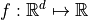from p functions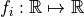.

We can do that:

• Case 1: using the tensor product of the functions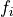,

• Case 2: by addition of the functions.

We need to implement both basic steps:

• Step 1: creation of the projection function: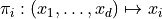,

• Step 2: creation of the composed function: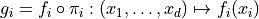.

## Step 1: Creation of the projection function¶

The projection function is defined by: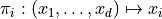We can do that using:

Method 1: We use the SymbolicFunction class.

import openturns as ot

def buidProjSymbolic(p, i):
# R^p --> R
# (x1, ..., xp) --> xi
inputVar = ot.Description.BuildDefault(p, 'x')
return ot.SymbolicFunction(inputVar, [inputVar[i]])

d = 2
all_projections = [buidProjSymbolic(d, i) for i in range(d)]
print('Input dimension = ', all_projections.getInputDimension(), 'Output dimension = ', all_projections.getOutputDimension())

Input dimension =  2 Output dimension =  1


Method 2: We use the LinearFunction class.

The function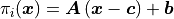.

def buildProjLinear(d, i):
# R^d --> R
# (x1, ..., xd) --> xi
matA = ot.Matrix(1, d)
matA[0, i] = 1.0
cVect = [0.0] * d
bVect = [0.0]
return ot.LinearFunction(cVect, bVect, matA)

all_projections = [buildProjLinear(d, i) for i in range(d)]


## Step 2: Creation of the composed function¶

The composed function is defined by: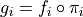defined by: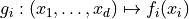We use the ComposedFunction class.

f1 = ot.SymbolicFunction(['x1'], ['x1^2'])
f2 = ot.SymbolicFunction(['x2'], ['3*x2'])
fi_list = [f1, f2]
all_g = [ot.ComposedFunction(f, proj) for (f, proj) in zip(fi_list, all_projections)]
print(all_g.getInputDimension(), all_g.getOutputDimension())

2 1


## Case 1: Tensor product¶

We want to build the functiondefined by: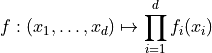As the operatorcan only be applied to functions sharing the same input space, we need to use the projection function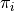and the functions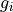all defined on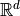.

def tensorProduct(factors):
prod = factors
for i in range(1, len(factors)):
prod = prod * factors[i]
return prod

f = tensorProduct(all_g)
print('input dimension =', f.getInputDimension())
print('output dimension =', f.getOutputDimension())
print('f([1.0, 2.0]) = ', f([1.0, 2.0]))

input dimension = 2
output dimension = 1
f([1.0, 2.0]) =  


## Case 2: Sum¶

We want to build the functiondefined by: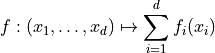We use the LinearCombinationFunction class.

coef = [1.0, 1.0]
f = ot.LinearCombinationFunction(all_g, [1.0] * len(all_g))
print('input dimension =', f.getInputDimension())
print('output dimension =', f.getOutputDimension())

input dimension = 2
output dimension = 1